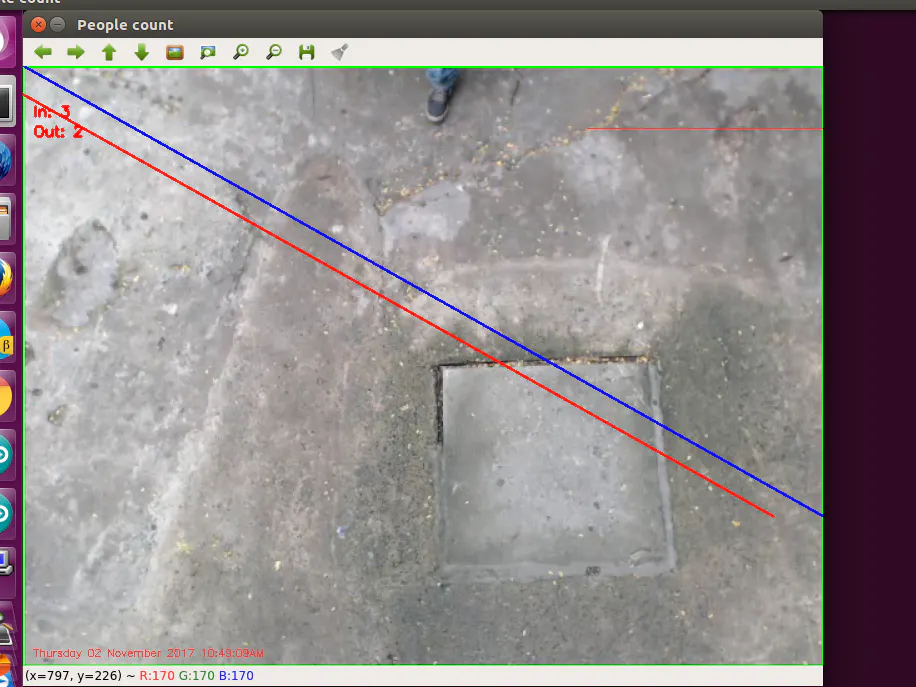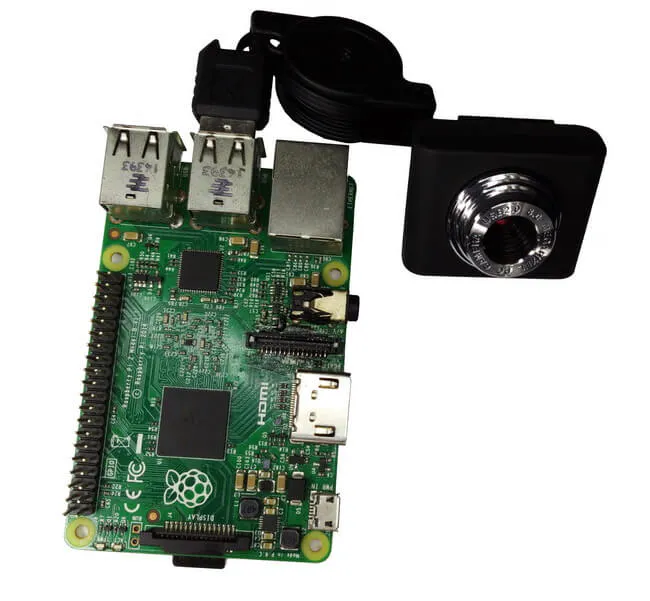# Person Counting System Using Opencv and Python

In this person counting system using Opencv and Python project, we are using one Raspberry Pi and one USB camera for this project.

IntermediateProtip3.5 hours3,541## Things used in this project

### Hardware componentsRaspberry Pi 3 Model B
×1

## Schematics

### Schematics

here is a Schematics## Code

### Project Code

Python
Here is a Project Code
```import argparse
import datetime
import imutils
import math

import cv2
import numpy as np

width = 800

textIn = 0
textOut = 0

def testIntersectionIn(x, y):

res = -450 * x + 400 * y + 157500
if((res >= -550) and  (res < 550)):
print (str(res))
return True
return False

def testIntersectionOut(x, y):
res = -450 * x + 400 * y + 180000
if ((res >= -550) and (res <= 550)):
print (str(res))
return True

return False

camera = cv2.VideoCapture(0)

firstFrame = None

while True:

text = "Unoccupied"

if not grabbed:
break

frame = imutils.resize(frame, width=width)
gray = cv2.cvtColor(frame, cv2.COLOR_BGR2GRAY)
gray = cv2.GaussianBlur(gray, (21, 21), 0)

if firstFrame is None:
firstFrame = gray
continue

frameDelta = cv2.absdiff(firstFrame, gray)
thresh = cv2.threshold(frameDelta, 25, 255, cv2.THRESH_BINARY)

thresh = cv2.dilate(thresh, None, iterations=2)
_, cnts, _ = cv2.findContours(thresh.copy(), cv2.RETR_EXTERNAL, cv2.CHAIN_APPROX_SIMPLE)

for c in cnts:
if cv2.contourArea(c) < 12000:
continue

(x, y, w, h) = cv2.boundingRect(c)
cv2.rectangle(frame, (x, y), (x + w, y + h), (0, 255, 0), 2)

cv2.line(frame, (width / 2, 0), (width, 450), (250, 0, 1), 2) #blue line
cv2.line(frame, (width / 2 - 50, 0), (width - 50, 450), (0, 0, 255), 2)#red line

rectagleCenterPont = ((x + x + w) /2, (y + y + h) /2)
cv2.circle(frame, rectagleCenterPont, 1, (0, 0, 255), 5)

if(testIntersectionIn((x + x + w) / 2, (y + y + h) / 2)):
textIn += 1

if(testIntersectionOut((x + x + w) / 2, (y + y + h) / 2)):
textOut += 1

if cv2.waitKey(1) & 0xFF == ord('q'):
break

cv2.putText(frame, "In: {}".format(str(textIn)), (10, 50),
cv2.FONT_HERSHEY_SIMPLEX, 0.5, (0, 0, 255), 2)
cv2.putText(frame, "Out: {}".format(str(textOut)), (10, 70),
cv2.FONT_HERSHEY_SIMPLEX, 0.5, (0, 0, 255), 2)
cv2.putText(frame, datetime.datetime.now().strftime("%A %d %B %Y %I:%M:%S%p"),
(10, frame.shape - 10), cv2.FONT_HERSHEY_SIMPLEX, 0.35, (0, 0, 255), 1)
cv2.imshow("Security Feed", frame)

camera.release()
cv2.destroyAllWindows()
```

## Credits

### Deligence Technologies

7 projects • 84 followers
IoT & Embedded Development team